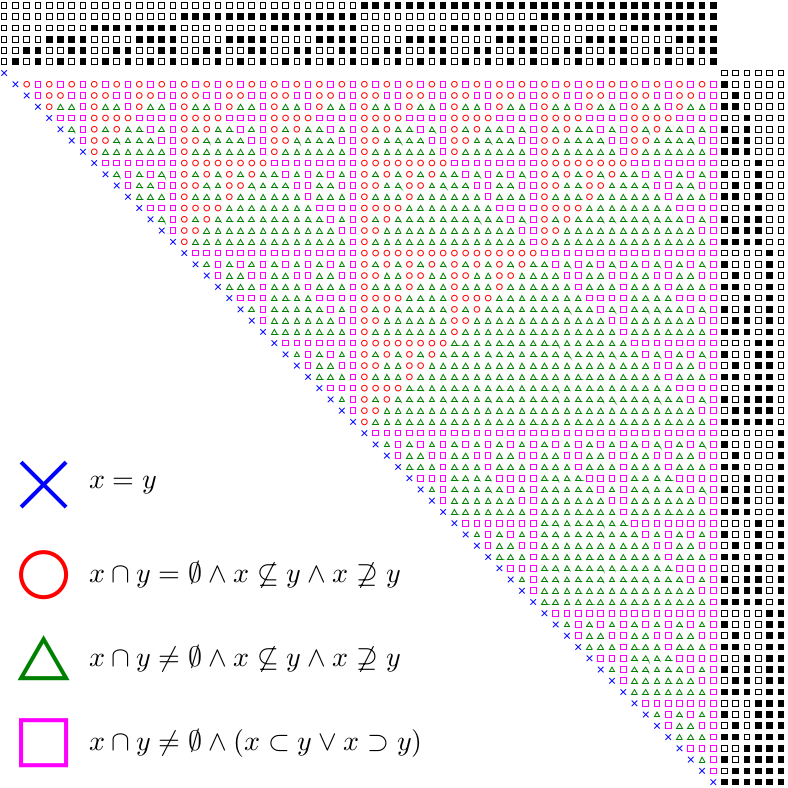# mathr / blog / #

## Rollover 2017Another year has arrived! 2017 is prime, so it occurs a lot in the Online Encyclopedia of Integer Sequences. I visualized one that isn't dependent on its primality: A134169. Haskell code below:

{-# LANGUAGE FlexibleContexts #-}
import Prelude hiding (null)

import Data.Bits (bit, finiteBitSize, testBit, (.&.))

import Data.Set (Set)
import qualified Data.Set as S

import Diagrams.Prelude hiding (intersection)
import Diagrams.Backend.PGF.CmdLine (B, defaultMain)

type Z = Int
type S = Int
type P = Set S

intersection :: S -> S -> S
intersection = (.&.)

isSubsetOf :: S -> S -> Bool
x isSubsetOf y = (x intersection y) == x

isProperSubsetOf :: S -> S -> Bool
x isProperSubsetOf y = (x isSubsetOf y) && x /= y

null :: S -> Bool
null x = x == 0

member :: Z -> S -> Bool
member i x = testBit x i

toList :: S -> [Z]
toList x = [ i | i <- [0 .. finiteBitSize x - 1], i member x ]

nset :: Z -> S
nset n = bit n - 1

npower :: Z -> P
npower n = S.fromList [0 .. bit n - 1]

data T = A | B | C | D

t :: S -> S -> Maybe T
t x y
| y > x = Nothing
|      null (x intersection y)  && not (x isSubsetOf y) && not (y isSubsetOf x) = Just A
| not (null (x intersection y)) && not (x isSubsetOf y) && not (y isSubsetOf x) = Just B
| not (null (x intersection y)) && ((x isProperSubsetOf y) || (y isProperSubsetOf x)) = Just C
| x == y = Just D
| otherwise = Nothing

label is x = [ square 2 # strokeP # lc black # fc (if i member x then black else white) # pad 2 | i <- is ]
xlabel s x = vcat $label (reverse$ toList s) x
ylabel s y = hcat $label ( toList s) y withEnvelope' :: Diagram B -> Diagram B -> Diagram B withEnvelope' = withEnvelope cell :: Maybe T -> Diagram B cell Nothing = withEnvelope' (square 2) mempty cell (Just A) = circle 1 # strokeP # lc red cell (Just B) = triangle 2 # centerXY # strokeP # lc green cell (Just C) = square 2 # strokeP # lc magenta cell (Just D) = (p2 (-1, -1) ~~ p2 (1, 1) atop p2 (1, -1) ~~ p2 (-1, 1)) # lc blue diagram n = lwL 0.25 . vcat$
( hcat $(++[withEnvelope' (ylabel s 0) mempty]) [ xlabel s x | x <- S.toList p ] ) : [ hcat$ (++[ylabel s y]) [ cell (t x y) # pad 2 | x <- S.toList p ] | y <- S.toList p ]
where
p = npower n
s = nset n

key a b c d = vcat
[ cell (Just D) # pad 2 ||| d
, cell (Just A) # pad 2 ||| a
, cell (Just B) # pad 2 ||| b
, cell (Just C) # pad 2 ||| c
] # scale 8

txt = alignedText 0 0.5

main1 :: Z -> IO ()
main1 n = defaultMain $let a = txt "$ x \\cap y   =   \\emptyset \\wedge x \\not\\subseteq y \\wedge x \\not\\supseteq y $" b = txt "$ x \\cap y \\neq \\emptyset \\wedge x \\not\\subseteq y \\wedge x \\not\\supseteq y $" c = txt "$ x \\cap y \\neq \\emptyset \\wedge \\left( x \\subset y \\vee x \\supset y \\right) $" d = txt "$ x = y $" m = 2^(n - 1) * (2^n - 1) + 1 count = txt$ "$" ++ show m ++ "$"
oeis = alignedText 0 0 "\\phantom{.} OEIS / A134169"
in  bg white . pad 1.1 . centerXY \$
alignBR (alignBL (diagram n # centerXY)
atop
alignBL (key a b c d # centerXY)
===
alignTL ((strutY 1.1 ||| count) # bold # scale 96))
atop
alignBR (rotate (90 @@ deg) (oeis # bold # scale 8))

main :: IO ()
main = main1 6

So, plans for the year ahead? I guess "continuity" sums it up - working on my projects, improving them, maybe finding cross-links between them. Playing around researching new things too. And peforming and exhibiting and presenting when opportunities arise. Concretely, here are a few project ideas:

cca
Blog about coupled cellular automata experiments, explore potential links with RDEX and BitBreeder projects, possible sonification.
clive
Look into the possibilities of cross-compilation and upload to Bela or other similar low-latency audio platform.
cmcms
Make year-rollover automatic instead of manual.
graphgrow
Blog about the updated Haskell GUI (GTK, OpenGL) with its Pd sonification. Think about how to present it as an installation, perhaps with touch screen. Also think about live performance possibilities.
hgmp
Battle-test my low-level Haskell bindings to GMP by writing FFI wrappers for my Mandelbrot-related C code. Will also require writing similar low-level bindings to MPFR, but which Haskell MPFR library to choose?
incidents
Try to make videos for another track or two at least. Blog about recent updates to the visualisation of the inflatable torus physics demo.
mandelbrot-book
Continue work on the reboot. Blog about it.
mandelbrot-*
Add new algorithms as I uncover them, document the existing code (with references to papers where possible), more examples.
mightymandel
Figure out how to merge with mandelbrot-perturbator.
monotone
Continue hacking at the code trying to speed it up enough to run in realtime on my hardware. Figure out how to profile the bottlenecks.
pool-party
Document and announce this water simulation demo.
tilda
Find a project or two for the EMFCamp 2016 badge.
unarchive